International
Tables for
Crystallography
Volume B
Reciprocal space
Edited by U. Shmueli

International Tables for Crystallography (2006). Vol. B. ch. 2.3, pp. 250-252   | 1 | 2 |

## Section 2.3.6.1. Introduction

M. G. Rossmanna* and E. Arnoldb

aDepartment of Biological Sciences, Purdue University, West Lafayette, Indiana 47907, USA, and  bCABM & Rutgers University, 679 Hoes Lane, Piscataway, New Jersey 08854-5638, USA
Correspondence e-mail:  mgr@indiana.bio.purdue.edu

#### 2.3.6.1. Introduction

| top | pdf |

The rotation function is designed to detect noncrystallographic rotational symmetry (see Table 2.3.6.1).The normal rotation function definition is given as (Rossmann & Blow, 1962)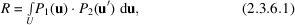whereandare two Pattersons and U is an envelope centred at the superimposed origins. This convolution therefore measures the degree of similarity, or overlap', between the two Pattersons whenhas been rotated relative toby an amount defined by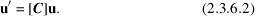The elements of [C] will depend on three rotation angles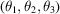. Thus, R is a function of these three angles. Alternatively, the matrix [C] could be used to express mirror symmetry, permitting searches for noncrystallographic mirror or glide planes.

 Table 2.3.6.1| top | pdf | Different types of uses for the rotation function
Type of rotation functionPattersons to be comparedPurposeSelf Unknown structure Unknown structure, same cell Finds orientation of noncrystallographic axes
Cross Unknown structure Unknown structure in different cell Finds relative orientation of unknown molecules
Cross Unknown structure Known structure in large cell to avoid overlap of self-Patterson vectors Determines orientation of unknown structure as preliminary to positioning and subsequent phasing with known molecule

The basic concepts were first clearly stated by Rossmann & Blow (1962), although intuitive uses of the rotation function had been considered earlier. Hoppe (1957b)had also hinted at a convolution of the type given by (2.3.6.1)to find the orientation of known molecular fragments and these ideas were implemented by Huber (1965).

Consider a structure of two identical units which are in different orientations. The Patterson function of such a structure consists of three parts. There will be the self-Patterson vectors of one unit, being the set of interatomic vectors which can be formed within that unit, with appropriate weights. The set of self-Patterson vectors of the other unit will be identical, but they will be rotated away from the first due to the different orientation. Finally, there will be the cross-Patterson vectors, or set of interatomic vectors which can be formed from one unit to another. The self-Patterson vectors of the two units will all lie in a volume centred at the origin and limited by the overall dimensions of the units. Some or all of the cross-Patterson vectors will lie outside this volume. Suppose the Patterson function is now superposed on a rotated version of itself. There will be no particular agreement except when one set of self-Patterson vectors of one unit has the same orientation as the self-Patterson vectors from the other unit. In this position, we would expect a maximum of agreement or overlap' between the two. Similarly, the superposition of the molecular self-Patterson derived from different crystal forms can provide the relative orientation of the two crystals when the molecules are aligned.

While it would be possible to evaluate R by interpolating inand forming the point-by-point product withwithin the volume U for every combination of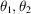and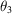, such a process is tedious and requires large computer storage for the Pattersons. Instead, the process is usually performed in reciprocal space where the number of independent structure amplitudes which form the Pattersons is about one-thirtieth of the number of Patterson grid points. Thus, the computation of a rotation function is carried out directly on the structure amplitudes, while the overlap definition (2.3.6.1)simply serves as a physical basis for the technique.

The derivation of the reciprocal-space expression depends on the expansion of each Patterson either as a Fourier summation, the conventional approach of Rossmann & Blow (1962), or as a sum of spherical harmonics in Crowther's (1972)analysis. The conventional and mathematically easier treatment is discussed presently, but the reader is referred also to Section 2.3.6.5for Crowther's elegant approach. The latter leads to a rapid technique for performing the computations, about one hundred times faster than conventional methods.

Let, omitting constant coefficients,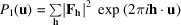and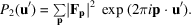From (2.3.6.2)it follows that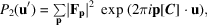and, hence, by substitution in (2.3.6.1)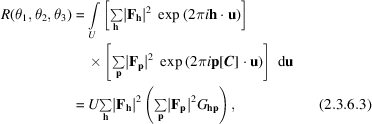where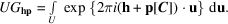When the volume U is a sphere,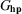has the analytical form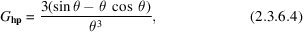where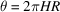and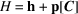. G is a spherical interference function whose form is shown in Fig. 2.3.6.1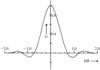Figure 2.3.6.1 | top | pdf |Shape of the interference function G for a spherical envelope of radius R at a distance H from the reciprocal-space origin. [Reprinted from Rossmann & Blow (1962).]

The expression (2.3.6.3)represents the rotation function in reciprocal space. If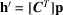in the argument of, then h′ can be seen as the point in reciprocal space to which p is rotated by [C]. Only for those integral reciprocal-lattice points which are close to h′ willbe of an appreciable size (Fig. 2.3.6.1). Thus, the number of significant terms is greatly reduced in the summation over p for every value of h, making the computation of the rotation function manageable.

The radius of integration R should be approximately equal to or a little smaller than the molecular diameter. If R were roughly equal to the length of a lattice translation, then the separation of reciprocal-lattice points would be about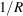. Hence, when H is equal to one reciprocal-lattice separation,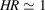, and G is thus quite small. Indeed, all terms with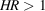might well be neglected. Thus, in general, the only terms that need be considered are those where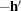is within one lattice point of h. However, in dealing with a small molecular fragment for which R is small compared to the unit-cell dimensions, more reciprocal-lattice points must be included for the summation over p in the rotation-function expression (2.3.6.3).

In practice, the equation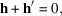that is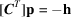or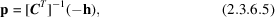determines p, given a set of Miller indices h. This will give a non-integral set of Miller indices. The terms included in the inner summation of (2.3.6.3)will be integral values of p around the non-integral lattice point found by solving (2.3.6.5).

Details of the conventional program were given by Tollin & Rossmann (1966)and follow the principles outlined above. They discussed various strategies as to which crystal should be used to calculate the first (h) and second (p) Patterson. Rossmann & Blow (1962)noted that the factor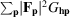in expression (2.3.6.3)represents an interpolation of the squared transform of the self-Patterson of the second (p) crystal. Thus, the rotation function is a sum of the products of the two molecular transforms taken over all the h reciprocal-lattice points. Lattman & Love (1970)therefore computed the molecular transform explicitly and stored it in the computer, sampling it as required by the rotation operation. A discussion on the suitable choice of variables in the computation of rotation functions has been given by Lifchitz (1983).

### ReferencesCrowther, R. A. (1972). The fast rotation function. In The molecular replacement method, edited by M. G. Rossmann, pp. 173–178. New York: Gordon & Breach.Google ScholarHoppe, W. (1957b). Die `Faltmolekülmethode' – eine neue Methode zur Bestimmung der Kristallstruktur bei ganz oder teilweise bekannter Molekülstruktur. Acta Cryst. 10, 750–751.Google ScholarHuber, R. (1965). Die automatisierte Faltmolekülmethode. Acta Cryst. 19, 353–356.Google ScholarLattman, E. E. & Love, W. E. (1970). A rotational search procedure for detecting a known molecule in a crystal. Acta Cryst. B26, 1854–1857.Google ScholarLifchitz, A. (1983). On the choice of the model cell and the integration volume in the use of the rotation function. Acta Cryst. A39, 130–139.Google ScholarRossmann, M. G. & Blow, D. M. (1962). The detection of sub-units within the crystallographic asymmetric unit. Acta Cryst. 15, 24–31.Google ScholarTollin, P. & Rossmann, M. G. (1966). A description of various rotation function programs. Acta Cryst. 21, 872–876.Google Scholar